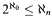Home

# Partitioning large vector spaces

## Extract

The theme of this paper is the generalization of theorems about partitions of the sets of points and lines of finite-dimensional Euclidean spaces ℝ d to vector spaces over ℝ of arbitrary dimension and, more generally still, to arbitrary vector spaces over other fields so long as these fields are not too big. These theorems have their origins in the following striking theorem of Sierpiński  which appeared a half century ago.

Sierpiński's Theorem. The Continuum Hypothesis is equivalent to: There is a partition {X, Y, Z} of3 such that if ℓ is a line parallel to the x-axis [respectively: y-axis, z-axis] then X [respectively: Yℓ, Z] is finite.

The history of this theorem and some of its subsequent developments are discussed in the very interesting article by Simms . Sierpiński's Theorem was generalized by Kuratowski  to partitions of ℝ n+2 into n + 2 sets obtaining an equivalence with. The geometric character that Sierpiński's Theorem and its generalization by Kuratowski appear to have is bogus, since the lines parallel to coordinate axes are essentially combinatorial, rather than geometric, objects. The following version of Kuratowski's theorem emphasizes its combinatorial character.

Kuratowski's Theorem. Let n < ω and A be any set. ThenA∣ ≤ ℵ n if and only if there is a partition P: A n+2n + 2 such that if in + 1 and ℓ is a line parallel to the i-th coordinate axis, then {x: P(x) = i} is finite.

Hide All

# Partitioning large vector spaces

## Metrics

### Full text viewsFull text views reflects the number of PDF downloads, PDFs sent to Google Drive, Dropbox and Kindle and HTML full text views.

Total number of HTML views: 0
Total number of PDF views: 0 *Loading metrics...

### Abstract viewsAbstract views reflect the number of visits to the article landing page.

Total abstract views: 0 *Loading metrics...

* Views captured on Cambridge Core between <date>. This data will be updated every 24 hours.

Usage data cannot currently be displayed